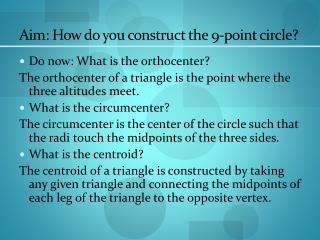Download PresentationAim: How do you construct the 9-point circle?

# Aim: How do you construct the 9-point circle?

Download Presentation## Aim: How do you construct the 9-point circle?

- - - - - - - - - - - - - - - - - - - - - - - - - - - E N D - - - - - - - - - - - - - - - - - - - - - - - - - - -
##### Presentation Transcript

1. Aim: How do you construct the 9-point circle? • Do now: What is the orthocenter? The orthocenter of a triangle is the point where the three altitudes meet. • What is the circumcenter? The circumcenter is the center of the circle such that the radi touch the midpoints of the three sides. • What is the centroid? The centroid of a triangle is constructed by taking any given triangle and connecting the midpoints of each leg of the triangle to the opposite vertex.

2. Construct a triangle ABC

3. Draw the circumcenter of the three sides, label them L,M, N

4. Sketch the orthocenter of triangle ABC Label the points of intersection as H Orthocenter = altitudes

5. Construct the midpoints of the segments : AH BH CH And label them as X, Y, Z

6. The 9-Point Circle